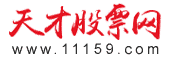首页　股票入门　看盘解盘　跟庄技巧　炒股技巧　选股技巧　买入技巧　卖出技巧　短线操作　K线　技术指标　分时图　均线　成交量　MACD　股票书籍　视频
 技术分析:　MACD　KDJ 股票投资:　股票入门

# 指标的变形和深化

asi指标是welles wilder所创的，他的本意是用股市中最高，最低，收盘和开盘四个数据构筑一条能真实反映市场运动的线，在飞狐中他的算法是：

LC:=REF(CLOSE,1);
AA:=ABS(HIGH-LC);
BB:=ABS(LOW-LC);
CC:=ABS(HIGH-REF(LOW,1));
DD:=ABS(LC-REF(OPEN,1));
R:=IF(AA>BB AND AA>CC,AA+BB/2+DD/4,IF(BB>CC AND BB>AA,BB+AA/2+DD/4,CC+DD/4));
X:=(CLOSE-LC+(CLOSE-OPEN)/2+LC-REF(OPEN,1));
SI:=16*X/R*MAX(AA,BB);
ASI:SUM(SI,0)；

a1:=abs(h-ref(c,1));
b1:=abs(l-ref(c,1));
c1:=abs(h-ref(l,1));
d1:=abs(ref(c,1)-ref(o,1));
e1:=c-ref(c,1);
f1:=c-o;
g1:=ref(c,1)-ref(o,1);
x:=e1+0.5*f1+g1;
k:=max(a1,b1);
r:=if(a1>b1 and a1>c1,a1+0.5*b1+0.25*d1,if(b1>a1 and b1>c1,b1+0.5*a1+0.25*d1,c1+0.25*d1));
l1:=3;
si:=16*(x/r)*(k/l1);
asi:=sum(si,0);

1:=abs(h-ref(c,1));
b1:=abs(l-ref(c,1));
c1:=abs(h-ref(l,1));
d1:=abs(ref(c,1)-ref(o,1));
e1:=c-ref(c,1);
f1:=c-o;
g1:=ref(c,1)-ref(o,1);
x:=e1+0.5*f1+g1;
k:=max(a1,b1);
r:=if(a1>b1 and a1>c1,a1+0.5*b1+0.25*d1,if(b1>a1 and b1>c1,b1+0.5*a1+0.25*d1,c1+0.25*d1));
l1:=3;
si:=16*(x/r)*(k/l1);
asi:=sum(si,20);

LC:=REF(CLOSE,1);
AA:=ABS(HIGH-LC);
BB:=ABS(LOW-LC);
CC:=ABS(HIGH-REF(LOW,1));
DD:=ABS(LC-REF(OPEN,1));
R:=IF(AA>BB AND AA>CC,AA+BB/2+DD/4,IF(BB>CC AND BB>AA,BB+AA/2+DD/4,CC+DD/4));
X:=(CLOSE-LC+(CLOSE-OPEN)/2+LC-REF(OPEN,1));
SI:=16*X/R*MAX(AA,BB);
ASI:=SUM(SI,20);
asi1:100*wma(asi,10) linethick1;
asi2:100*wma(asi,3)linethick1;

LC:=REF(CLOSE,1);
AA:=ABS(HIGH-LC);
BB:=ABS(LOW-LC);
CC:=ABS(HIGH-REF(LOW,1));
DD:=ABS(LC-REF(OPEN,1));
R:=IF(AA>BB AND AA>CC,AA+BB/2+DD/4,IF(BB>CC AND BB>AA,BB+AA/2+DD/4,CC+DD/4));
X:=(CLOSE-LC+(CLOSE-OPEN)/2+LC-REF(OPEN,1));
SI:=16*X/R*MAX(AA,BB);
ASI:=SUM(SI,20);
asi1:100*wma(asi,10) linethick1;
asi2:100*wma(asi,3)linethick1;
pp:(if(asi1>0 and asi2>0,pow(abs(asi1*asi2),0.5),-pow(abs(asi1*asi2),0.5)))linethick1;
qq:(asi1+asi2)/2 linethick1

1 k线有几种类型呢？

2 股市的运动不同用什么判断呢？

3 我们判断一般问题的方法是什么呢？

1 物体运动，我们判断他用速度，加速度。股市中我也试图找到与这两个量相应的东西，我称作趋势力度和力度的东西。打个比方，昨天股价2块钱，今天4块，明天5块，从昨天到明天力度是增大的，但趋势是减小的，昨天到今天趋势力度是2块，今天到明天趋势力度是1块，这就是判断股市运动的一种方法。由这个想法我想到k线应该有这几种类型：
2 a 阳线趋势力度强，力度强
b 阴线……
c
d等8种情况，这8种情况反映了股市运动的不同状态。研究不同状态是我们研究问题的方法吗？
3 我们研究问题，从一般到特殊是一种，分类判断也是一种很重要的方法。太极两仪再到八卦，推而演64卦在我看来就是一种分类研究的方法分开研究，研究透了，在和起来，整个问题也就解决了。

asi:=(asi1-ref(asi1,1))/ref(asi,1);
pp:=asi*ref(asi,1)*ref(asi,2)*ref(asi,3)*ref(asi,4);
qq:=pow(abs(pp),1/5);
xx:=if(pp>0,qq,-qq);
asi是变化率，下面是平滑的过程。

ASIp:=SUM(SI,20);
li:=100*wma(asip,15) ;
xiao:=100*wma(asip,7);

 相关文章:
 图解教程: 浏览器不支持嵌入式框架，或被配置为不显示嵌入式框架。
 推荐教程 K线图入门与实战 成交量入门与实战 分时图入门与实战 MACD入门与实战 跟庄入门与实战 选股入门与实战 均线入门与实战 KDJ入门与实战
 热点教程　　 　　　　　　　　　　更多
 股票书籍　　 　　　　　　　　　　更多
 Copyright 2019 天才股票网 www.11159.com 联系我们